Next: B. Some Tools from Up: Dissertation Hajdin Ceric Previous: 5. Summary and Outlook

# A. Vacancies in Solids

A perfect crystal at equilibrium at zero temperature is comprised of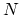lattice sites, each with a lattice atom. At any finite temperature, a small population of monovacancies,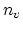, forms spontaneously, such that the total number of lattice sites increases to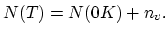The spontanuos formation of point defect above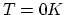occurs, because the Gibbs free energy is lowered slightly for a crystal containing a small concentration of vacancies. A vacancy can be created by removing an atom from its substitional site and placing it on the surface. The energy required to do this under constant pressure conditions is the entalphy of formation of a vacancy,. According to classical theory for silicon, the formation of vacancy requires the breaking of four bonds to remove a lattice atom, and the forming of two new bonds to place this atom on the surface. New bonds are formed between dangling bonds of the four neighbors of the vacancy, so that the neighbors undergo relaxation and distortion. Then,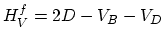(A.1)

where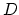is the bond dissociation energy,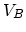is the energy gained by bonding between the neighbors of the vacancy, and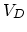is the energy associated with deformation of all other bonds.
The Gibbs free energy of a crystal withvacancies is,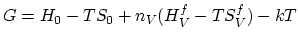ln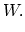(A.2)

The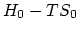represents the Gibbs free energy of the perfect vacancy-free crystal and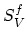is the disorder induced by lattice vibration. The last term on the right side of (A.2) is the configurational entropy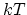ln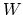, where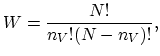(A.3)

i.e., W is the number of distinguishable ways of distributing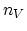vacancies onlattice sites. The equilibrium concentration of lattice vacancies,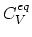, forming at a particular temperature is defined as,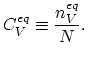(A.4)

This fractional concentration occurs when the Gibbs free energy (A.2) is minimized with respect to, keepingconstant,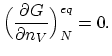(A.5)

Based on this condition after a few steps of algebra we obtain the important result,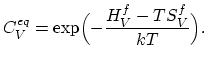(A.6)

This equation can be generalized to any type of defect.
In semiconductors defects can exhibit various charge states. For instance in silicon, four charge states have been reported for the vacancy: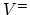,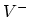,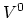and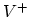. Under extrinsic condition, not only does the relative population of the different charge states change depending on the position of the electro-chemical potential (Fermi level), but the total concentration of the point defect in the crystal also changes. It is possible to directly calculate the concentration of charged defects and express it in terms of the Fermi level, considering reactions involving the charged defects which satisfy the charge neutrality constraint. Let us consider the reaction involving the ionization of the neutral vacancyinto a positive vacancy,and an electron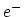,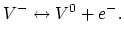(A.7)

For this reaction, the free energy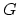is a minimum at equilibrium with respect toand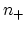, the number of neutral and positively charged vacancies, respectively. This implies that the equilibrium condition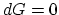can be written as,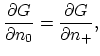(A.8)

which simply expresses the equality of chemical potentials. Solving (A.8) allows the relative concentrations of the vacancies to be written as,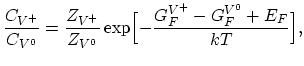(A.9)

where,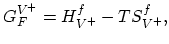(A.10)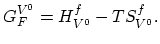(A.11)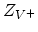,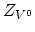are internal degeneracies and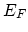is the Fermi level. Equation (A.9) is completely general and allows the concentration of any charged vacancy to be obtained in terms of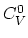and the Fermi level.Next: B. Some Tools from Up: Dissertation Hajdin Ceric Previous: 5. Summary and Outlook

H. Ceric: Numerical Techniques in Modern TCAD